# Using ISERROR with VLOOKUP in Excel

In this tutorial, we will look at how to use the ISERROR with VLOOKUP in Excel to handle all kinds of errors productively.

VLOOKUP is one of the most confusing Excel functions plagued with many issues. Whichever table you are looking in, #N/A errors are a common sight, with #NAME and #VALUE also appearing now and then. Using VLOOKUP with ISERROR can help you catch all possible errors and handle them in a way most appropriate to your situation.

## Why is VLOOKUP giving an error?

The most common error in VLOOKUP formulas is #N/A occurring when a lookup value is not found. This may happen because of different reasons:

• The lookup value does not exist in the lookup array.
• The lookup value is misspelt.
• There are leading or trailing spaces in the lookup value or lookup column.
• The lookup column is not the leftmost column of the table array.

Besides, you can run into a #VALUE! error, e.g. when the lookup value contains more than 255 characters. In case there is a spelling error in the function's name, a #NAME? error will appear.

For full reference, please see our earlier post on Why Excel VLOOKUP is not working.

## IF ISERROR VLOOKUP formula to replace errors with custom text

To disguise all possible errors that can be triggered by VLOOKUP, you can place it inside of the IF ISERROR formula like this:

IF(ISERROR(VLOOKUP(…)), "text_if_error", VLOOKUP(…))

As an example, let's pull the names of the subjects in which the students of group A failed tests:

`=VLOOKUP(A3, \$D\$3:\$E\$9, 2, FALSE)`

As the result, you are getting a bunch of #N/A errors, which might create an impression that the formula is corrupt.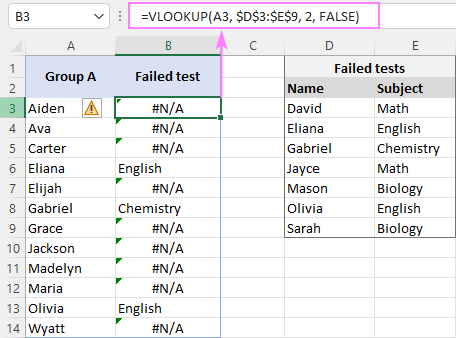In truth, these errors just indicate that some of the lookup values (A3:A14) are not found in the lookup list (D3:D9). To clearly convey that idea, nest your VLOOKUP formula in the IF ISERROR construction:

`=IF(ISERROR(VLOOKUP(A3, \$D\$3:\$E\$9, 2, FALSE)), "No", VLOOKUP(A3, \$D\$3:\$E\$9, 2, FALSE))`

This will catch errors and return your custom text message: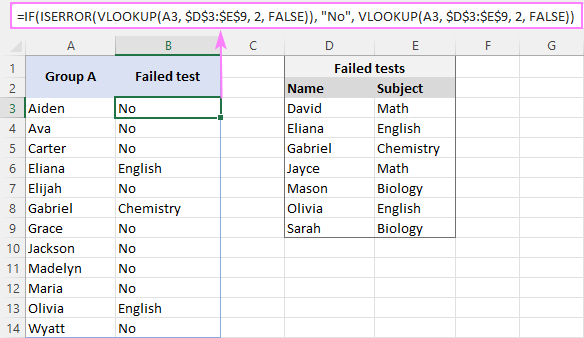Tips and notes:

• The main advantage of this formula is that it works nicely in all versions of Excel 2000 through Excel 365. In modern versions, simpler and more compact alternatives are available.
• The ISERROR function catches absolutely all errors, such as #N/A, #NAME, #VALUE, etc. In case you wish to display a custom message only when a lookup value is not found (#N/A error), use the IF ISNA VLOOKUP (in all versions) or IFNA VLOOKUP (in Excel 2013 and later).

## ISERROR VLOOKUP to return blank cell if error

To have a blank cell when an error occurs, get your formula to return an empty string ("") instead of a custom text:

IF(ISERROR(VLOOKUP(…)), "", VLOOKUP(…))

In our case, the formula takes this form:

`=IF(ISERROR(VLOOKUP(A3, \$D\$3:\$E\$9, 2, FALSE)), "", VLOOKUP(A3, \$D\$3:\$E\$9, 2, FALSE))`

The result is exactly as expected - a blank cell if the student's name is not found in the lookup table.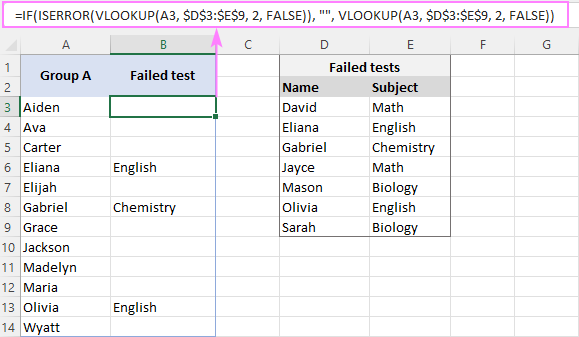Tip. In a similar manner, you can replace VLOOKUP errors with zeros, dashes or any other character you like. Just use the desired character in place of an empty string.

## IF ISERROR VLOOKUP Yes/No formula

In some situation, you may be looking for something but instead of pulling the matches just want to return Yes (or some other text if the lookup value is found) and No (if the lookup value is not found). To have it done, you can use this generic formula:

IF(ISERROR(VLOOKUP(…)), "text_if_not_found", "text_if_found")

In our sample dataset, suppose you wish to know which students failed a test and which did not. To accomplish this, serve the already familiar ISERROR VLOOKUP formula to the logical test of IF and tell it to output "No" if the value is not found (ISERROR VLOOKUP returns TRUE), "Yes" if found (ISERROR VLOOKUP returns FALSE):

`=IF(ISERROR(VLOOKUP(A3, \$D\$3:\$E\$9, 2, FALSE)), "No", "Yes")`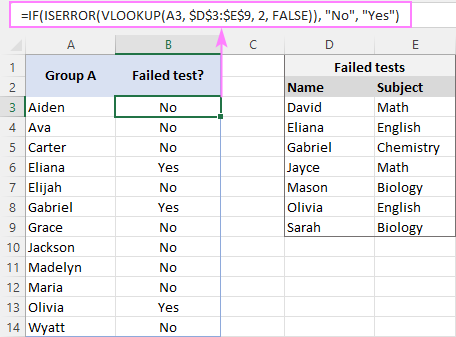## ISERROR VLOOKUP alternatives

The IF ISERROR combination is the oldest time proven technique to Vlookup without errors in Excel. Over time, new functions evolved, providing easier ways to perform the same task. Below, we will discuss other possible solutions and when each is best to be applied.

### IFERROR VLOOKUP

Available in Excel 2007 and higher

Beginning with version 2007, Excel has a special function, named IFERROR, to check a formula for errors and return your own text (or run an alternative formula) if any error is detected.

IFERROR(VLOOKUP(…), "text_if_error")

The real-life formula is as follows:

`=IFERROR(VLOOKUP(A3, \$D\$3:\$E\$9, 2, FALSE), "No")`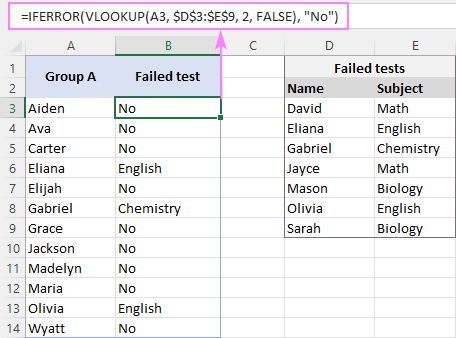At first sight, it looks like a shorter analogue of the IF ISERROR VLOOKUP formula. However, there is an essential difference:

• IFERROR VLOOKUP assumes that you always want the result of VLOOKUP if it isn't an error.
• IF ISERROR VLOOKUP lets you specify what to return if an error and what if no error.

For more details, please see Using IFERROR with VLOOKUP in Excel.

### IF ISNA VLOOKUP

Works in Excel 2000 and later

In situation when you want to trap only #N/A without catching any other errors, the ISNA function comes in handy. The syntax is the same as that of IF ISERROR VLOOKUP:

IF(ISNA(VLOOKUP(…)), "text_if_error", VLOOKUP(…))

But under certain circumstances, this seemingly identical formula may produce different results:

`=IF(ISNA(VLOOKUP(A3, \$D\$3:\$E\$9, 2, FALSE)), "No", VLOOKUP(A3, \$D\$3:\$E\$9, 2, FALSE))`

In the image below, cell A13 contains plenty of trailing spaces because of which the total length of the lookup value exceeds 255 characters. As the result, the formula triggers a #VALUE! error, drawing your attention to that cell and encouraging to investigate the reasons. ISERROR VLOOKUP would return "No" in this case, which would only obscure the issue and deliver an absolutely wrong result.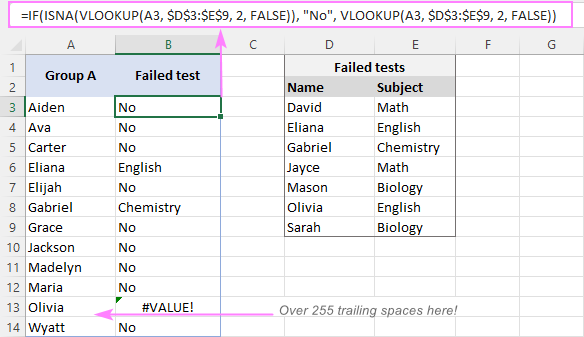When to use:

This formula works beautifully in a situation when you want to display some text only when a lookup value is not found and do not want to mask underlying problems with the VLOOKUP formula itself, e.g. when the function's name is mistyped (#NAME?) or the full path to the lookup workbook is not specified (#VALUE!).

### IFNA VLOOKUP

Available in Excel 2013 and higher

It is a modern replacement of the IF ISNA combination that lets you handle #N/A errors in an easier way.

IFNA(VLOOKUP(…), "text_if_error")

Here's a shorthand equivalent of our IF ISNA VLOOKUP formula:

`=IFNA(VLOOKUP(A3, \$D\$3:\$E\$9, 2, FALSE), "No")`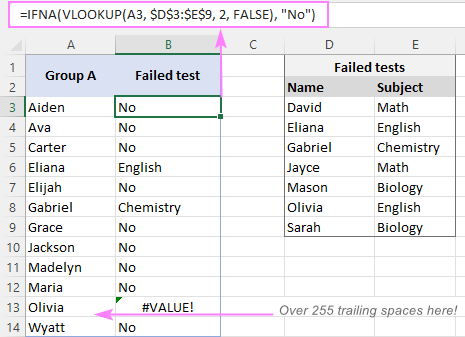When to use:

It's an ideal solution to trap and handle #N/A errors in modern versions of Excel (2013 - 365).

For full details, see Excel IFNA function.

### XLOOKUP

Supported in Excel 2021 and Excel 365

Due to its inbuilt "if error" functionality, the XLOOKUP function is the easiest way to look up without #N/A errors in Excel. Simply, type your user-friendly text in the optional 4th argument named if_not_found.

For example:

`=XLOOKUP(A3, \$D\$3:\$D\$9, \$E\$3:\$E\$9, "No")`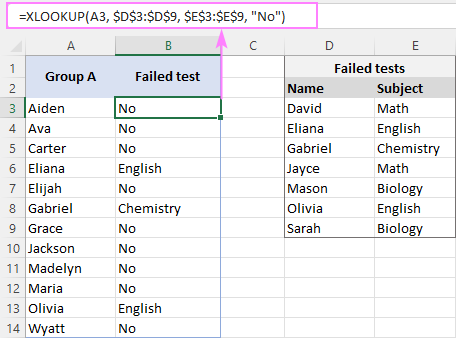Limitation: It only catches #N/A errors, ignoring other types.